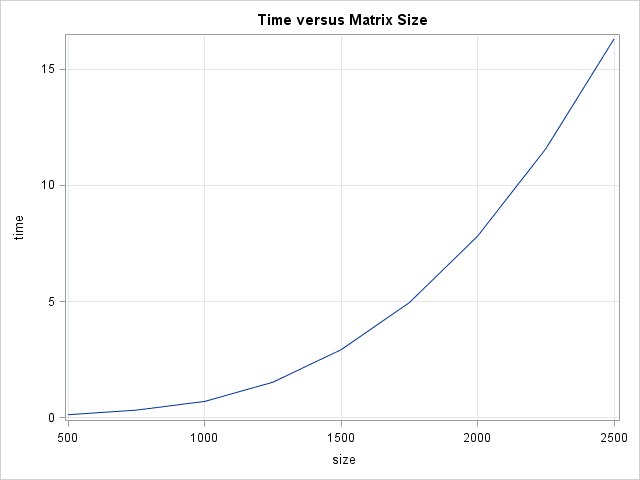As my colleague Margaret Crevar recently wrote, it is useful to know how long SAS programs take to run. Margaret and others have written about how to use the SAS FULLSTIMER option to monitor the performance of the SAS system. In fact, SAS distributes a macro that enables you to parse SAS logs to extract performance and timing information.

But for researchers who are developing custom algorithms in the SAS/IML language, there is a much simpler way to monitor performance. You can use the TIME function in Base SAS to find out (to within about 15 milliseconds) how long it takes for a SAS/IML function or set of statements to run. I use this function often to assess how an algorithm scales with the size of the input data. The TIME function returns the time of day, so if you call it twice and compute the difference in times, you get the time (in seconds) that elapsed between calls.

For example, suppose that you need to compute eigenvalues of a large symmetric matrix. You might be interested in knowing how the algorithm scales with the size of the (square) input matrix. The following SAS/IML program uses the SQRVECH function to create symmetric matrices of size 500, 1000, ..., 2500. For each matrix the TIME function is called just before and immediately after a call to the EIGVAL function, which computes the eigenvalues of the matrix. The elapsed time is plotted against the size of the matrix:

```proc iml; size = T(do(500, 2500, 250)); /* 500, 1000, ..., 2500 */ time = j(nrow(size), 1); /* allocate room for results */ call randseed(12345); do i = 1 to nrow(size); n = size[i]; r = j(n*(n+1)/2, 1); call randgen(r, "uniform"); /* generate random elements */ A = sqrvech(r); /* form symmetric matrix */   t0 = time(); /* save the current time */ val = eigval(A); /* put computation here */ time[i] = time() - t0; /* compute elapsed time */ end;   title "Time versus Matrix Size"; call series(size, time) grid={x y};```The line plot (click to enlarge) shows the timing of the eigenvalue computation on square matrices of varying sizes. The computation is very fast when the matrices are less than 1000 x 1000, but takes longer as the matrix grows. For a 2500 x 2500 matrix, the computation takes about 15 seconds.

You can also use the TIME function to compare the performance of two or more different algorithms. For example, you can compare the performance of solving linear systems to help you write efficient programs.

You can also use this technique to time how long SAS procedures take to run: You can use the SUBMIT/ENDSUBMIT statements to call any SAS procedure, which means you can "drive" the performance analysis from the SAS/IML language. This technique is much easier than parsing SAS logs!

Incidentally, the distribution of the eigenvalues for a matrix with random elements that are drawn from a given distribution is a fascinating topic that is part of "random matrix theory." For a peek into this beautiful mathematical topic, see the article "The curious case of random eigenvalues", which discusses the symmetric matrices that I used in today's article. For unsymmetric matrices, the eigenvalues can be complex, and the distribution of the eigenvalues in the complex plane makes beautiful images.

For more details about timing computations and assessing the performance of algorithms, see Chapter 15 of Statistical Programming with SAS/IML Software.

ShareDistinguished Researcher in Computational Statistics

Rick Wicklin, PhD, is a distinguished researcher in computational statistics at SAS and is a principal developer of SAS/IML software. His areas of expertise include computational statistics, simulation, statistical graphics, and modern methods in statistical data analysis. Rick is author of the books Statistical Programming with SAS/IML Software and Simulating Data with SAS.

1.•